# HSSlive: Plus One & Plus Two Notes & Solutions for Kerala State Board

## BSEB Class 10 Maths Chapter 6 Triangles Ex 6.3 Textbook Solutions PDF: Download Bihar Board STD 10th Maths Chapter 6 Triangles Ex 6.3 Book AnswersBSEB Class 10 Maths Chapter 6 Triangles Ex 6.3 Textbook Solutions PDF: Download Bihar Board STD 10th Maths Chapter 6 Triangles Ex 6.3 Book Answers

BSEB Class 10th Maths Chapter 6 Triangles Ex 6.3 Textbooks Solutions and answers for students are now available in pdf format. Bihar Board Class 10th Maths Chapter 6 Triangles Ex 6.3 Book answers and solutions are one of the most important study materials for any student. The Bihar Board Class 10th Maths Chapter 6 Triangles Ex 6.3 books are published by the Bihar Board Publishers. These Bihar Board Class 10th Maths Chapter 6 Triangles Ex 6.3 textbooks are prepared by a group of expert faculty members. Students can download these BSEB STD 10th Maths Chapter 6 Triangles Ex 6.3 book solutions pdf online from this page.

## Bihar Board Class 10th Maths Chapter 6 Triangles Ex 6.3 Books Solutions

 Board BSEB Materials Textbook Solutions/Guide Format DOC/PDF Class 10th Subject Maths Chapter 6 Triangles Ex 6.3 Chapters All Provider Hsslive

## How to download Bihar Board Class 10th Maths Chapter 6 Triangles Ex 6.3 Textbook Solutions Answers PDF Online?

2. Click on the Bihar Board Class 10th Maths Chapter 6 Triangles Ex 6.3 Answers.
3. Look for your Bihar Board STD 10th Maths Chapter 6 Triangles Ex 6.3 Textbooks PDF.
4. Now download or read the Bihar Board Class 10th Maths Chapter 6 Triangles Ex 6.3 Textbook Solutions for PDF Free.

Find below the list of all BSEB Class 10th Maths Chapter 6 Triangles Ex 6.3 Textbook Solutions for PDF’s for you to download and prepare for the upcoming exams:

## BSEB Bihar Board Class 10th Maths Solutions Chapter 6 Triangles Ex 6.3

Question 1.
State which pairs of triangle in the figures, are similar. Write the similarity criterion used by you for answering the question and also write the pairs of similar triangles in the symbolic form :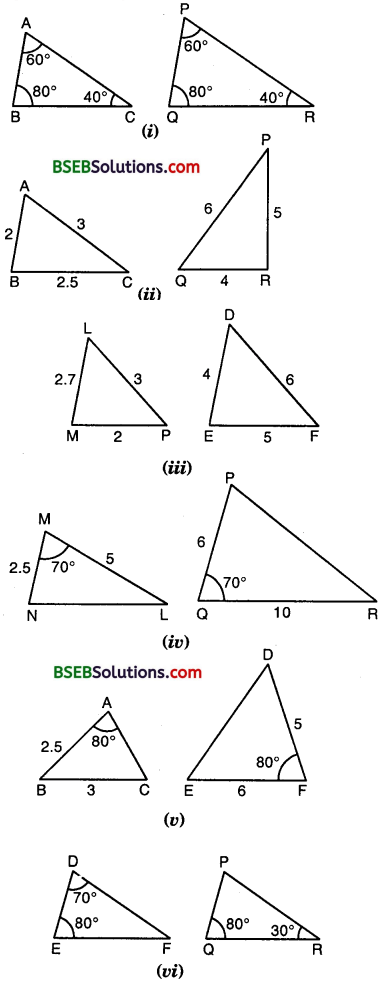Solution:
(i) In ∆s ABC and PQR, we observe that
∠A = ∠P = 60°, ∠B = ∠Q = 80° and ∠C = ∠R = 40°. By AAA criterion of similarity,
∆ ABC ~ ∆ PQR.

(ii) In ∆s ABC and PQR, we observe that
𝐴𝐵𝑄𝑅 = 𝐵𝐶𝑅𝑃 = 𝐶𝐴𝑃𝑄 = 12
∴By SSS criterion of similarity, ∆ ABC ~ ∆ QRP.

(iii) In ∆s LMP and DEF, we observe that the ratio of the sides of these triangles are not equal.
[ 𝑀𝑃𝐷𝐸 = 𝐿𝑃𝐷𝐹 but it is not equal to 𝐿𝑀𝐸𝐹 ]
So, the two triangles are not similar.

(iv) In ∆s MNL and QPR, we observe that
∠M = ∠Q = 70°
But, 𝑀𝑁𝑃𝑄 ≠ 𝑀𝐿𝑄𝑅
These two triangles are not similar as they do not satisfy SAS criterion of similarity.

(v) In ∆s ABC and FDE, we have
∠A = ∠F = 80°
But, 𝐴𝐵𝐷𝐸 ≠ 𝑀𝐿𝑄𝑅 [∵ AC is not given]
DE DF
So, by SAS criterion’ of similarity, these two triangles are not similar.

(vi) In ∆s DEF and PQR, we have :
∠D = ∠P = 70° [∵∠P = 180° – 80° – 30° = 70°]
∠E = ∠Q = 80°
So, by AAA criterion of similarity,
∆ DEF ~ ∆ PQR.

Question 2.
In the figure,∆ ODC ~ ∆ OBA, ∠BOC = 125° and ∠CDO = 70°. Find ∠DOC, ∠DCO and ∠OAB.
Solution:
Since BD is a line and OC is a ray on it,
∴∠DOC + ∠BOC = 180°
So, ∠DOC + 125° = 180°
or ∠DOC = 180°
In ∆ CDO, we have :
∠CDO + ∠DOC + ∠DCO = 180°
or 70° + 55° + ∠DCO = 180°
or ∠DCO = 180° – 125° = 55°
It is given that ∆ ODC ~ A OBA
∴ ∠ODC = ∠OBA, ∠OCD = ∠OAB
or ∠OBA = 70° and ∠OAB = 55°
Hence, ∠DOC = 55° ∠DCO = 55° and ∠OAB = 55°

Question 3.
Diagonals AC and BD of a trapezium ABCD with | DC intersect each other at the point O. Using asimilarity criterion for two triangles, show that 𝑂𝐴𝑂𝐶 = 𝑂𝐵𝑂𝐷
Solution:
Given : ABCD is a trapezium in which AB || DC.
To prove : 𝑂𝐴𝑂𝐶 = 𝑂𝐵𝑂𝐷
Proof:
In As OAB and OCD, we have :
∠AOB = ∠COD [Vert. opp. ∠s]
∠OAB = ∠OCD [Alternate ∠s]
and ∠OBA = ∠ODC [Alternate ∠s]∴ By AAA criterion of similarity,
∆ OAB ~ ∆ OCD
Hence 𝑂𝐴𝑂𝐶 = 𝑂𝐵𝑂𝐷 [ ∵ In case of two similar triangles the ratio of their corresponding sides are equal]

Question 4.
In the figure, 𝑄𝑅𝑄𝑆 = 𝑄𝑇𝑃𝑅 and ∠1 = ∠2. Show that ∆ PQS ~ ∆ TQR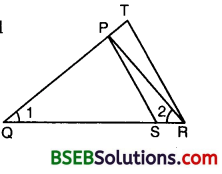Solution: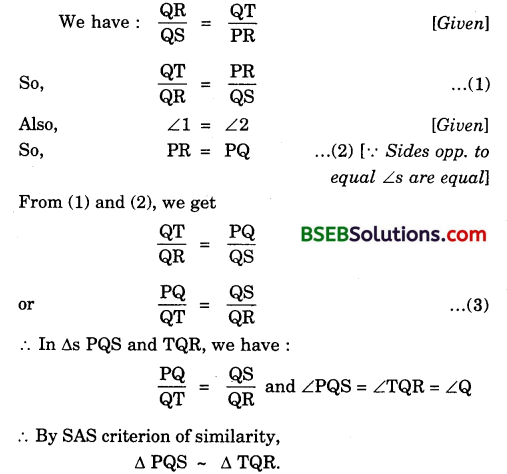Question 5.
S and T are points on sides PR and QR of ∆ PQR such that ∠P = ∠RTS. Show that ∆ RPQ ~ ∆ RTS.
Solution:
In ∆s RPQ and RTS, we have :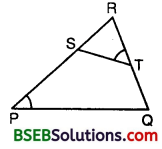∠RPQ = ∠RTS [Given]
∠PRQ = ∠TRS [Common]
∴ By AA criterion of similarity,
∆ RPQ ~ ∆ RTS.

Question 6.
In the figure, if ∆ ABE ≅ ∆ ACD, show that ∆ ADE ~ ∆ ABC.Solution:
It is given that ∆ ABE ≅ ∆ ACD
AB = AC [∵ Corresponding parts of congruent triangles are equal]
So, 𝐴𝐵𝐴𝐷 = 𝐴𝐶𝐴𝐸 = or 𝐴𝐵𝐴𝐶 = 𝐴𝐷𝐴𝐸
∴ In As ADE and ABC, we have :
𝐴𝐵𝐴𝐶 = 𝐴𝐷𝐴𝐸 [ ∵of (1) ]
and ∠BAC = ∠DAE [common]
Thus, by SAS criterion of similarity,

Question 7.
In the figure, altitudes AD and CE of ∆ ABC intersect each other at the point P.
Show that: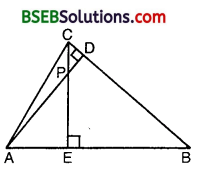(i) ∆AEP ~ ∆ CDP
(ii) ∆ ABD ~ ∆ CBE
(iii) ∆ AEP ~ ∆ ADB
(iv) ∆ PDC ~ ∆ BEC
Solution:
(i) In ∆s AEP and CDP, we have :
∠AEP = ∠CDP = 90° [∵ CE ⊥ AB and AD ⊥ BC]
∠APE = ∠CPD [Vert. opp. ∠s]
∴By AA criterion of similarity, we have :
∆ AEP ~ ∆ CDP.

(ii) In ∆s ∆BD and CBE, we have :
∠ABD = ∠CBE [Common angle]
∴By AA criterion of similarity, we have :
∆ ABD – ∆ CBE.

(iii) In ∆s AEP and ADB, we have :
∠AEP = ∠ADB = 90° [∵AD ⊥ BC and CE ⊥ AB]
∠PAE = ∠DAB [Common angle]
∴By AA criterion of similarity, we have :

(iv) In ∆s PDC and BEC, we have :
∠PDC = ∠BEC = 90° [∵ AD ⊥ BC and CE ⊥ AB]
∠PCD = ∠ECB [Common angle]
∴ By AA criterion of similarity, we have :
∆ PDC – ∆ BEC.

Question 8.
E is a point on the side ALD produced of a parallelogram ABCD and BE intersects CD at F. Show that ∆ ABE ~ ∆ CFB.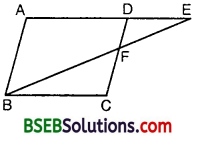Solution:
In ∆s ABE and CFB, we have:
∠AEB = ∠CBF [Alt. ∠s]
∠A = ∠C [Opp. ∠s of a gm]
∴By AA criterion of similarity, B we have :
∆ ABE – ∆ CFB.

Question 9.
In the figure, ABC and AMP are two right triangles, right angled at B and M respectively. Prove that :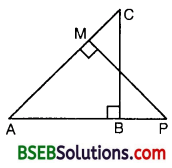(i) ∆ ABC ~ ∆ AMP
(ii) 𝐶𝐴𝑃𝐴 = 𝐵𝐶𝑀𝑃
Solution:
(i) In ∆s ABC and AMP, we have :
∠ABC = ∠AMP = 90° [Given]
and ∠BAC = ∠MAP [Common ∠s]
∴By AA criterion of similarity, we have :
∆ ABC – ∆ AMP.

(ii) We have :
∆ ABC ~ ∆ AMP [As proved above]
So, 𝐶𝐴𝑃𝐴 = 𝐵𝐶𝑀𝑃 [In similar triangles, correspondings sides are proportional.

Question 10.
CD and GH are respectively the bisectors of ∠ACB and ∠EGF such that D and H lie on sides AB and FE of A ABC and ∆ EFG respectively. If ∆ ABC ~ ∆ FEG, show that :
(i) 𝐶𝐷𝐺𝐻 = 𝐴𝐶𝐹𝐺
(ii) ∆ DCB ~ ∆ HGE
(iii) ∆ DCA ~ ∆ HGF
Solution:We have : ∆ ABC ~ ∆ FEG
So, ∠A = ∠F
and ∠C = ∠G
∴12 = 12 ∠G
or ∠1 = ∠3 and ∠2 = ∠4 … (2)
[∵ CD and GH are bisectors of ∠C and ∠G respectively]
∴ In As DCA and HGF, we have :
∠A = ∠F
∠2 = ∠4 [From (2)]
∴By AA criterion of similarity, we have :
∆ DCA – ∆ HGF
which proves the (iii) part.
We have : ∆ DCA ~ ∆ HGF [As proved above]
So, 𝐴𝐶𝐹𝐺 = 𝐶𝐷𝐺𝐻 or 𝐶𝐷𝐺𝐻 = 𝐴𝐶𝐹𝐺
which proves the (i) part.
In As DCB and HGE, we have :
∠1 = ∠3 [From (2)]
∠B = ∠E [∵ ∆ ABC ~ ∆ FEG]
∴By AA criterion of similarity, we have :
∆ DCB ~ ∆ HGE
which proves the (ii) part.

Question 11.
In the figure, E is a point on side CB produced of an isosceles triangle ABC with AB = AC. If AD ⊥ BC and EF ⊥ AC, prove that ∆ ABD ~ ∆ ECF.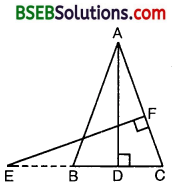Solution:
Here, ∆ ABC is isosceles with AB = AC.
∴ ∠B = ∠C
In ∆s ABD and ECF, we have :
∠ABD = ∠ECF [∵ ∠B = ∠C]
∠ADB = ∠EFC = 90° [∵ AD ⊥ BC and EF ⊥ AC]
∴ By AA criterion of similarity,
∆ ABD ~ ∆ ECF.

Question 12.
Sides AB and BC and median AD of a triangle ABC are respectively proportional to sides PQ and QR and median PM of ∆ PQR (see figure). Show that ∆ ABC ~ ∆ PQR.Solution:
Given : AD is the median of ∆ ABC and PM is the median of ∆ PQR such that
𝐴𝐵𝑃𝑄 = 𝐵𝐶𝑄𝑅 = 𝐴𝐷𝑃𝑀
To prove : ∆ ABC ~ ∆ PQR∴ By SSS criterion of similarity, we have :
∆ ABD ~ ∆ PQM
So, ∠B = ∠Q [Similar ∆s have corresponding ∠s equal]
Also, [Given]
𝐴𝐵𝑃𝑄 = 𝐵𝐶𝑄𝑅 [Given]
∴ By SAS criterion of similarity, we have :
∆ ABC ~ ∆ PQR.

Question 13.
D is a point on the side BC of a triangle ABC such that ∠ADC = ∠BAC. Show that CA² = CB.CD.
Solution:
In ∆s ABC and DAC, we have :
∠BAC = ∠ADC and ∠C = ∠C
∴ By AA criterion of similarity, we have :∆ ABC – ∆ DAC
So, 𝐴𝐵𝐷𝐴 = 𝐵𝐶𝐴𝐶 = 𝐴𝐶𝐷𝐶
Now, from 𝐵𝐶𝐴𝐶 = 𝐴𝐶𝐷𝐶
𝐶𝐵𝐶𝐴 = 𝐶𝐴𝐶𝐷
or CA² = CB x CD.

Question 14.
Sides AB and AC and median AD of a triangle ABC are respectively proportional to sides PQ and PR and median PM of another triangle PQR. Show that ∆ ABC ~ ∆ PQR.
Solution:
Produce AD to a point E such that AD = DE and PM to a point N such that PM = MN (see figure). Join CE and RN.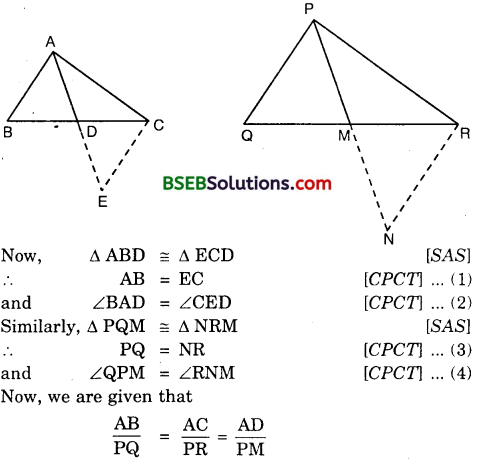So, from (1) and (3), we have :Question 15.
A vertical pole of length 6 m casts a shadow 4 m long on the ground and at the same time a tower casts a shadow 28 m long. Find the height of the tower.
Solution: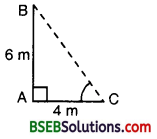Let AB be the vertical pole B and AC be its shadow. Also, let DE be the vertical tower and DF be its shadow. 6 m Join BC and EF. Let DE = x metres.
We have : AB = 6 m, AC = 4 m and DF = 28 m.
In As ABC and DEF, we have :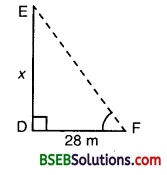∠A = ∠D = 90°,
and ∠C = ∠F
[∵ Each is the angular elevation of the sun]
∴ By AA criterion of similarity,
∆ ABC ~ ∆ DEF
or 𝐴𝐵𝐷𝐸 = 𝐴𝐶𝐷𝐹
So, 6𝑥 = 428 or 6𝑥 = 17
or x = 6 x 7 = 42.
Hence, the height of the tower is 42 metres.

Question 16.
If AD and PM are medians of triangles ABC and PQR respectively, where ∆ ABC ~ ∆ PQR, prove 𝐴𝐵𝑃𝑄 = 𝐴𝐷𝑃𝑀
Solution:
Given : AD and PM are the medians of As ABC and PQR respectively, where
∆ ABC ~ ∆ PQR
To Prove : 𝐴𝐵𝑃𝑄 = 𝐴𝐷𝑃𝑀
Proof: In As ABD and PQM, we have :## Bihar Board Class 10th Maths Chapter 6 Triangles Ex 6.3 Textbooks for Exam Preparations

Bihar Board Class 10th Maths Chapter 6 Triangles Ex 6.3 Textbook Solutions can be of great help in your Bihar Board Class 10th Maths Chapter 6 Triangles Ex 6.3 exam preparation. The BSEB STD 10th Maths Chapter 6 Triangles Ex 6.3 Textbooks study material, used with the English medium textbooks, can help you complete the entire Class 10th Maths Chapter 6 Triangles Ex 6.3 Books State Board syllabus with maximum efficiency.

## FAQs Regarding Bihar Board Class 10th Maths Chapter 6 Triangles Ex 6.3 Textbook Solutions

#### Can we get a Bihar Board Book PDF for all Classes?

Yes you can get Bihar Board Text Book PDF for all classes using the links provided in the above article.

## Important Terms

Bihar Board Class 10th Maths Chapter 6 Triangles Ex 6.3, BSEB Class 10th Maths Chapter 6 Triangles Ex 6.3 Textbooks, Bihar Board Class 10th Maths Chapter 6 Triangles Ex 6.3, Bihar Board Class 10th Maths Chapter 6 Triangles Ex 6.3 Textbook solutions, BSEB Class 10th Maths Chapter 6 Triangles Ex 6.3 Textbooks Solutions, Bihar Board STD 10th Maths Chapter 6 Triangles Ex 6.3, BSEB STD 10th Maths Chapter 6 Triangles Ex 6.3 Textbooks, Bihar Board STD 10th Maths Chapter 6 Triangles Ex 6.3, Bihar Board STD 10th Maths Chapter 6 Triangles Ex 6.3 Textbook solutions, BSEB STD 10th Maths Chapter 6 Triangles Ex 6.3 Textbooks Solutions,
Share: